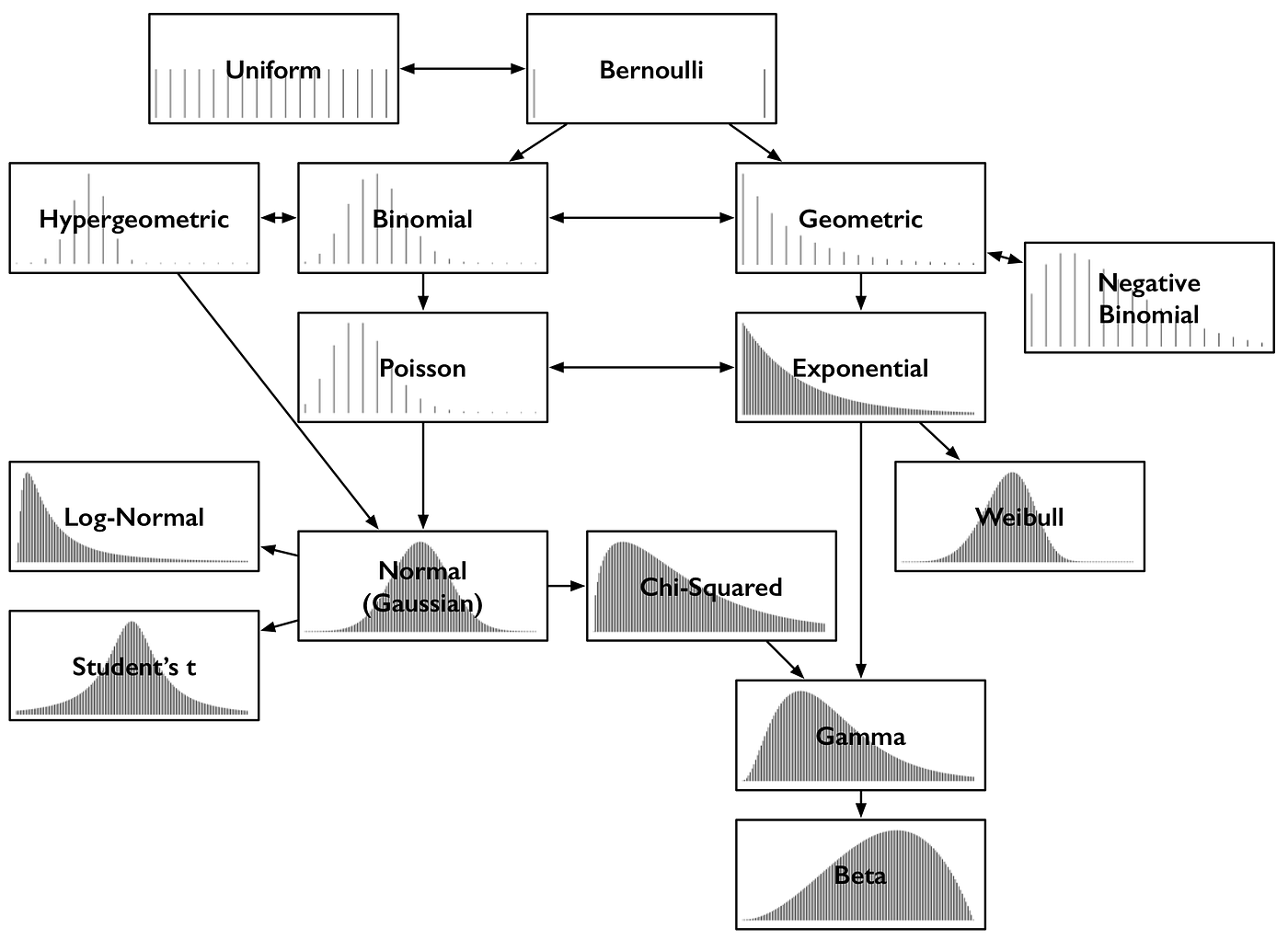# Now, What’s a Probability Distribution?

Things happen all the time: dice are rolled, it rains, buses arrive. After the fact, the specific outcomes are certain: the dice came up 3 and 4, there was half an inch of rain today, the bus took 3 minutes to arrive. Before, we can only talk about how likely the outcomes are. Probability distributions describe what we think the probability of each outcome is, which is sometimes more interesting to know than simply which single outcome is most likely. They come in many shapes, but in only one size: probabilities in a distribution always add up to 1.Common probability distributions and some key relationships

# Bernoulli and Uniform

You met the Bernoulli distribution above, over two discrete outcomes — tails or heads. Think of it, however, as a distribution over 0 and 1, over 0 heads (i.e. tails) or 1 heads. Above, both outcomes were equally likely, and that’s what’s illustrated in the diagram. The Bernoulli PDF has two lines of equal height, representing the two equally-probable outcomes of 0 and 1 at either end.

# Binomial and Hypergeometric

The binomial distribution may be thought of as the sum of outcomes of things that follow a Bernoulli distribution. Toss a fair coin 20 times; how many times does it come up heads? This count is an outcome that follows the binomial distribution. Its parameters are n, the number of trials, and p, the probability of a “success” (here: heads, or 1). Each flip is a Bernoulli-distributed outcome, or trial. Reach for the binomial distribution when counting the number of successes in things that act like a coin flip, where each flip is independent and has the same probability of success.

# Poisson

What about the count of customers calling a support hotline each minute? That’s an outcome whose distribution sounds binomial, if you think of each second as a Bernoulli trial in which a customer doesn’t call (0) or does (1). However, as the power company knows, when the power goes out, 2 or even hundreds of people can call in the same second. Viewing it as 60,000 millisecond-sized trials still doesn’t get around the problem — many more trials, much smaller probability of 1 call, let alone 2 or more, but, still not technically a Bernoulli trial. However, taking this to its infinite, logical conclusion works. Let n go to infinity and let p go to 0 to match so that np stays the same. This is like heading towards infinitely many infinitesimally small time slices in which the probability of a call is infinitesimal. The limiting result is the Poisson distribution.

# Geometric and Negative Binomial

From simple Bernoulli trials arises another distribution. How many times does a flipped coin come up tails before it first comes up heads? This count of tails follows a geometric distribution. Like the Bernoulli distribution, it’s parameterized by p, the probability of that final success. It’s not parameterized by n, a number of trials or flips, because the number of failure trials is the outcome itself.

# Exponential and Weibull

Back to customer support calls: how long until the next customer calls? The distribution of this waiting time sounds like it could be geometric, because every second that nobody calls is like a failure, until a second in which finally a customer calls. The number of failures is like the number of the seconds that nobody called, and that’s almost the waiting time until the next call, but almost isn’t close enough. The catch this time is that the sum will always be in whole seconds, but this fails to account for the wait within that second until the customer finally called.

# Normal, Log-Normal, Student’s t, and Chi-squared

The normal distribution, or Gaussian distribution, is maybe the most important of all. Its bell shape is instantly recognizable. Like e, it’s a curiously particular entity that turns up all over, from seemingly simple sources. Take a bunch of values following the same distribution — any distribution — and sum them. The distribution of their sum follows (approximately) the normal distribution. The more things that are summed, the more their sum’s distribution matches the normal distribution. (Caveats: must be a well-behaved distribution, must be independent, only tends to the normal distribution.) The fact that this is true regardless of the underlying distribution is amazing.

# Gamma and Beta

At this point, if you’re talking about chi-squared anything, then the conversation has gotten serious. You are likely talking to actual statisticians, and you may want to excuse yourself at this point, because things like the gamma distribution may come up. It is a generalization of both the exponential and chi-squared distributions. More like the exponential distribution, it is used as a sophisticated model of waiting times. For example, the gamma distribution comes up when modeling the time until the next n events occur. It appears in machine learning as the “conjugate prior” to a couple distributions.

# The Beginning of Wisdom

Probability distributions are something you can’t know too much about. The truly interested should check out this incredibly detailed map of all univariate distributions. Hopefully, this anecdotal guide gives you the confidence to appear knowledgeable and with-it in today’s tech culture. Or at least, a way to detect, with high probability, when you should find a less nerdy cocktail party.

--

--